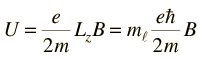## > The Zeeman effect and its use in Remote Magnetometry for stars

The spin-orbit interaction can be considered as a case of an "internal magnetic field" affecting atomic energy levels. In this section, the influence of external magnetic fields is examined.

When a magnetic dipole is placed in a magnetic field it will experience a torque. Let us analyse this.

Let us consider a “singlet state”. In quantum mechanics, a “singlet state” (term related to the notion of “single spectral line”, is a system where the spin or spin angular momentum is zero and therefore the total angular momentum is equal to the orbital angular momentum. (It is noted that if the spin angular momenta can sum vectorially to zero, the spin is zero.)

As mentioned at https://en.wikipedia.org/wiki/Magnetic_moment, the magnetic (dipole) moment of a magnet is a quantity that determines the torque it will experience in an external magnetic field.

We know how to calculate the torque exerted by the magnetic field on a coil with current I, surface A and n loops. It is described at the link http://hyperphysics.phy-astr.gsu.edu/hbase/magnetic/magmom.html#c2. We will use the same approach.

A magnetic source in general is inherently a dipole source that can be visualized as a coil, or let us say a loop with current I and area A as shown at the picture below from link http://hyperphysics.phy-astr.gsu.edu/hbase/magnetic/magmom.html#c1.

Figure 2: Image from hyperphysics.phy-astr.gsu.edu

http://hyperphysics.phy-astr.gsu.edu/hbase/magnetic/magmom.html#c1.

The magnetic (dipole) moment can be considered to be a vector μ with a value equal to I * A and a direction perpendicular to the current loop in the right-hand-rule direction. The torque is given by τ = μ * B.

Due to this, a "capacity for doing work" is generated as a result of the magnetic field. The "capacity for doing work" or "potential for doing work" (cf. common expression "this candidate for the job has got potential!") is translated to the notion of "potential energy" as mentioned at http://hyperphysics.phy-astr.gsu.edu/hbase/pegrav.html#pe. In particular, due to the fact that this potential is linked to a magnetic field, this is termed "magnetic potential energy" (cf. http://hyperphysics.phy-astr.gsu.edu/hbase/magnetic/magpot.html#c1).

The magnetic potential energy U of a magnetic (dipole) moment μ due to the presence of a magnetic field B is given by:

U = - μ * B

The magnetic potential energy is expressed as a scalar product (μ and B are vectors) and it implies that the torque that is exerted tends to line up/to align the magnetic moment with the magnetic field B, so this represents its lowest energy configuration.

The magnetic dipole moment is due to the orbital angular momentum. The relation associating them is:

μorb = - (e/2m) * L

If we consider the magnetic field in the z-direction, given that Lz =mℓ * h, we will haveU = - e/(2m) * L* B = mℓ * eh/(2m) * B

This potential energy U will correspond to the change of the energy in the system due to the torque from the magnetic field. Therefore, we will continue with the term ΔΕ.

ΔΕ= m * μΒ * Β  where μΒ is the Bohr magnetron

How many values of m (magnetic quantum number) do we have?

For instance for ℓ = 2:        m = −2, −1, 0, 1, 2,

In general we have 2ℓ +1 values of m (cf. for ℓ =2 we have 5 values of mℓ above )

This means that 5 different energetic levels will be produced (or can be attributed). In other words, there will be "splitting" in five different levels.

As mentioned at the link http://hyperphysics.phy-astr.gsu.edu/hbase/quantum/sodzee.html#c2 "using the vector model for total angular momentum, the splitting is seen to produce one level for each possible value of the z-component of the total angular momentum J".

It is noted that in this case the splitting will be uniform.

If we have five lines, what are the different energetic jumps that can be made? That is related to the Δm or change of m.

The change of mℓ can be -1, 0 or +1 and there will be only three transition energies:

E0 + ehB/2mℓ,

E0

E0 - ehB/2m

By dividing the change in energy by h, we can find the change of frequency of the emitted spectral line. Therefore, the change of frequency will be:

eB/2m

0

eB/2m

Figure 1: From the gross structure of the atom to the fine and hyperfine structure. The spin-orbit interaction is linked to the fine structure and the Zeeman interaction to the hyperfine structure and magnetic sublevels.